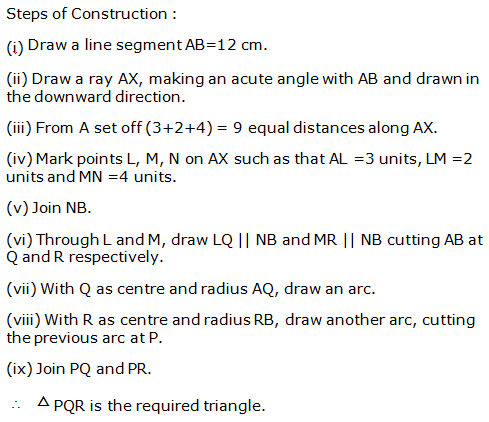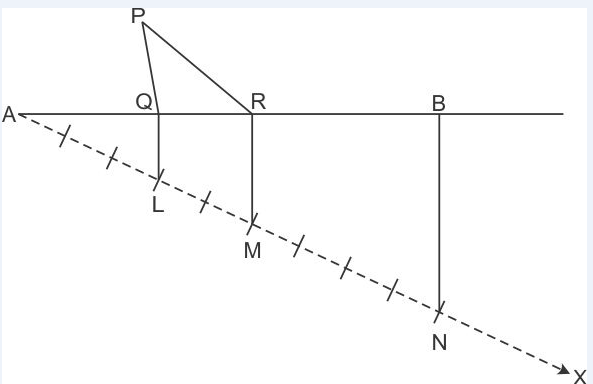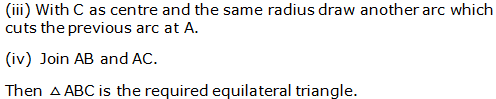# R S AGGARWAL AND V AGGARWAL Solutions for Class 9 Maths Chapter 13 - Geometrical Constructions

Page / Exercise

## Chapter 13 - Geometrical Constructions Exercise Ex. 13

Question 1

Draw a line segment AB = 5.6 cm and draw its perpendicular bisector. Measure the length of each part.

Solution 1

Steps of construction:

1. Draw line segment AB = 5.6 cm
2. With A as centre and radius more than half of AB, draw two arcs, one on each side of AB.
3. With B as centre and the same radius as in step 2, draw arcs cutting the arcs drawn in the previous step at P and Q respectively.
4. Join PQ to intersect AB at M.

Thus, PQ is the required perpendicular bisector of AB.AM = BM = 2.8 cm

Question 2

Construct a ΔABC in which BC = 4.5 cm, B = 45° and AB + AC = 8 cm. Justify your construction.

Solution 2

Steps of construction:

1. Draw BC = 4.5 cm
2. Draw CBX = 45°
3. From ray BX, cut-off line segment BD equal to AB + AC, i.e. 8 cm.
4. Join CD.
5. Draw the perpendicular bisector of CD meeting BD at A.
6. Join CA to obtain the required triangle ABC.Justification:

Clearly, A lies on the perpendicular bisector of CD.

Now, BD = 8 cm

BA + AD = 8 cm

AB + AC = 8 cm

Hence, ΔABC is the required triangle.

Question 3

Construct a ΔABC in which AB = 5.8 cm, B = 60° and BC + CA = 8.4 cm. Justify your construction.

Solution 3

Steps of construction:

1. Draw AB = 5.8 cm
2. Draw ABX = 60°
3. From ray BX, cut off line segment BD = BC + CA = 8.4 cm.
5. Draw the perpendicular bisector of AD meeting BD at C.
6. Join AC to obtain the required triangle ABC.Justification:

Clearly, C lies on the perpendicular bisector of AD.

CA = CD

Now, BD = 8.4 cm

BC + CD = 8.4 cm

BC + CA = 8.4 cm

Hence, ΔABC is the required triangle.

Question 4

Construct a ΔABC in which BC = 6 cm, B = 30° and AB - AC = 3.5 cm. Justify your construction.

Solution 4

Steps of construction:

1. Draw base BC = 6 cm
2. Construct CBX = 30°
3. From ray BX, cut off line segment BD = 3.5 cm (= AB - AC)
4. Join CD.
5. Draw the perpendicular bisector of CD which cuts BX at A.
6. Join CA to obtain the required triangle ABC.Justification:

Since A lies on the perpendicular bisector of CD.

Now, BD = 3.5 cm

AB - AD = 3.5 cm

AB - AC = 3.5 cm

Hence, ΔABC is the required triangle.

Question 5

Construct a ΔABC in which base AB = 5 cm, A = 30° and AC - BC = 2.5 cm. Justify your construction.

Solution 5

Steps of construction:

1. Draw base AB = 5 cm
2. Construct BAX = 30°
3. From ray AX, cut off line segment AD = 2.5 cm (= AC - BC)
4. Join BD.
5. Draw the perpendicular bisector of BD which cuts AX at C.
6. Join BC to obtain the required triangle ABC.Justification:

Since C lies on the perpendicular bisector of BD.

CD = BC

AC - CD = 2.5 cm

AC - BC = 2.5 cm

Hence, ΔABC is the required triangle.

Question 6

Construct awhose perimeter is 12 cm and the lengths of whose sides are in the ratio 3:2:4.

Solution 6Question 7

Construct a triangle whose perimeter is 10.4 cm and the base angles are 45° and 120°.

Solution 7

Steps of Construction:

1. Draw a line segment PQ = 10.4 cm.
2. Construct a 45° angle and bisect it to get NPQ.
3. Construct a 120° angle and bisect it to get MQP.
4. Let the rays PN and QM intersect at A.
5. Construct the perpendicular bisectors of PA and QA, to intersect PQ at B and C respectively.
6. Join AB and AC.

So, ΔABC is the required triangle.Question 8

Construct a ΔABC whose perimeter is 11.6 cm and the base angles are 45° and 60°.

Solution 8

Steps of Construction:

1. Draw a line segment PQ = 11.6 cm.
2. Construct a 45° angle and bisect it to get NPQ.
3. Construct a 60° angle and bisect it to get MQP.
4. Let the rays PN and QM intersect at A.
5. Construct the perpendicular bisectors of PA and QA, to intersect PQ at B and C respectively.
6. Join AB and AC.

So, ΔABC is the required triangle.Question 9

In each of the following cases, given reasons to show that the construction of ΔABC is not possible:

AB = 6 cm, A = 40° and (BC + AC) = 5.8 cm.

Solution 9

Given,

AB = 6 cm, A = 40° and (BC + AC) = 5.8 cm

We know that the sum of any two sides of a triangle is greater than the third side.

Here, we find that BC + AC < AB

Hence, construction of triangle ABC with given measurements is not possible.

Question 10

In each of the following cases, given reasons to show that the construction of ΔABC is not possible:

AB = 7 cm, A = 50° and (BC - AC) = 8 cm.

Solution 10

Given,

AB = 7 cm, A = 50° and (BC - AC) = 8 cm

We know that the sum of any two sides of a triangle is greater than the third side.

That is,

AB + AC > BC

AB + AC - AC > BC - AC

AB > BC - AC

Here, we find that AB < BC - AC

Hence, construction of triangle ABC with given measurements is not possible.

Question 11

In each of the following cases, given reasons to show that the construction of ΔABC is not possible:

BC = 5 cm, B = 80°, ∠C = 50° and A = 60°.

Solution 11

Given,

BC = 5 cm, B = 80°, ∠C = 50° and A = 60°

We know that the sum of the measures of three angles of a triangle is 180°.

Here, we find that

A + B + C = 60° + 80° + 50° = 190° > 180°

Hence, construction of triangle ABC with given measurements is not possible.

Question 12

In each of the following cases, given reasons to show that the construction of ΔABC is not possible:

AB = 4 cm, BC = 3 cm and AC = 7 cm.

Solution 12

Given,

AB = 4 cm, BC = 3 cm and AC = 7 cm

We know that the sum of any two sides of a triangle is greater than the third side.

Here, we find that AB + BC = AC

Hence, construction of triangle ABC with given measurements is not possible.

Question 13

Construct an angle of 67.5° by using the ruler and compasses.

Solution 13

Steps for Construction:

1. Draw a line XY.

2. Take a point A on XY.

3. With A as the centre, draw a semi-circle, cutting XY at P and Q.

4. Construct YAC = 90°.

5. Draw the bisector AB of XAC. Then YAB = 135°.

6. Draw the bisector AM of YAB. Then YAM = 67.5°.Question 14

Construct a square of side 4 cm.

Solution 14

Steps of Construction:

1. Draw a line segment PQ = 4 cm.

2. Construct QPX = 90° and PQY = 90°.

3. Cut an arc PS = 4 cm and QR = 4 cm. Join SR.

So, PQRS is the required square.Question 15

Draw an angle of 80° with the help of a protractor and bisect it. Measure each part of the bisected angle.

Solution 15

Steps of construction:

1. Draw ray OB.

2. With the help of protractor construct an angle AOB of measure 80°.

3. With centre O and convenient radius draw an arc cutting sides OA and OB at Q and P respectively.

4. With centre Q and radius more than half of PQ, draw an arc.

5. With centre P and the same radius, as in the previous step, draw another arc intersecting the arc drawn in the previous step at R.

6. Join OR and produce it to form ray OX.

Then, OX is the required bisector of AOB.AOX = BOX = 40°

Question 16

Construct a right triangle whose one side is 3.5 cm and the sum of the other side and the hypotenuse is 5.5 cm.

Solution 16

Steps of construction:

1. Draw BC = 3.5 cm
2. Draw CBX = 90°
3. From ray BX, cut off line segment BD = AB + AC = 5.5 cm.
4. Join CD.
5. Draw the perpendicular bisector of CD meeting BD at A.
6. Join AC to obtain the required triangle ABC.Question 17

Construct a ΔABC in which B = 45°, C = 60° and the perpendicular from the vertex A to base BC is 4.5 cm.

Solution 17

Steps of construction:

1. Draw any line XY.
2. Take any point P on XY and draw PQ XY.
3. Along PQ, set off PA = 4.5 cm.
4. Through A, draw LM XY.
5. Construct LAB = 45° and MAC = 60°, meeting XY at B and C respectively.

Then, ΔABC is the required triangle.Question 18

Construct an angle ofusing ruler and compasses and bisect it.

Solution 18

Step of Construction:

(i) Draw a line segment OA.

(ii) With O as centre and any suitable radius draw an arc, cutting OA at B.

(iii) With B as centre and the same radius cut the previously drawn arc at C.

(iv) With C as centre and the same radius cut the arc at D.

(v) With C as centre and the radius more than half CD draw an arc.

(vi) With D as centre and the same radius draw another arc which cuts the previous arc at E.

(vii) Join ENow,AOE =900

(viii) Now with B as centre and radius more than half of CB draw an arc.

(iv) With C as centre and same radius draw an arc which cuts the previousat F.

(x) Join OF.

(xi)F is the bisector of rightAOE.Question 19

Construct each of the following angles, using ruler and compasses:

75°

Solution 19

Steps of construction:

1. Draw a line segment PQ.
2. With centre P and any radius, draw an arc which intersects PQ at R.
3. With centre R and same radius, draw an arc which intersects previous arc at S.
4. With centre S and same radius, draw an arc which intersects arc in step 2 at T.
5. With centres T and S and radius more than half of TS, draw arcs intersecting each other at U.
6. Join PU which intersects arc in step 2 at V.
7. With centres V and S and radius more than half of VS, draw arcs intersecting each other at W.
8. Join PW.

WPQ = 75°Question 20

Construct each of the following angles, using ruler and compasses:

37.5°

Solution 20

Steps of construction:

1. Draw a line segment PQ.
2. With centre P and any radius, draw an arc which intersects PQ at R.
3. With centre R and same radius, draw an arc which intersects previous arc at S.
4. With centre S and same radius, draw an arc which intersects arc in step 2 at T.
5. With centres T and S and radius more than half of TS, draw arcs intersecting each other at U.
6. Join PU which intersects arc in step 2 at V.
7. With centres V and S and radius more than half of VS, draw arcs intersecting each other at W.
8. Join PW. WPQ = 75°
9. Bisect WPQ.

Then, ZPQ = 37.5°Question 21

Construct each of the following angles, using ruler and compasses:

135°

Solution 21

Steps of construction:

1. Draw a line segment AB and produce BA to point C.
2. With centre A and any radius, draw an arc which intersects AC at D and AB at E.
3. With centres D and E and radius more than half of DE, draw two arcs which intersect each other at F.
4. Join FA which intersects the arc in step 2 at G.
5. With centres G and D and radius more than half of GD, draw two arcs which intersect each other at H.
6. Join HA.

Then, HAB = 135°Question 22

Construct each of the following angles, using ruler and compasses:

105°

Solution 22

Steps of construction:

1. Draw a line segment PQ.
2. With centre P and any radius, draw an arc which intersects PQ at R.
3. With centre R and same radius, draw an arc which intersects previous arc at S.
4. With centre S and same radius, draw an arc which intersects arc in step 2 at T.
5. With centres T and S and radius more than half of TS, draw arcs intersecting each other at U.
6. Join PU which intersects arc in step 2 at V.
7. Now taking T and V as centres, draw arcs with radius more than half the length TV.
8. Let these arcs intersect each other at W.
9. Join PW, which is the required ray making 105°with the given ray PQ.

Then, WPQ = 105°Question 23

Construct each of the following angles, using ruler and compasses:

22.5°

Solution 23

Steps of construction:

1. Draw a line segment AB.
2. With centre A and any radius, draw an arc which intersects AB at C.
3. With centre C and same radius, draw an arc which intersects previous arc at D.
4. With centre D and same radius, draw an arc which intersects arc in step 2 at E.
5. With centres E and D and radius more than half of ED, draw arcs intersecting each other at F.
6. Join AF which intersects arc in step 2 at G.
7. Now taking G and C as centres, draw arcs with radius more than half the length GC.
8. Let these arcs intersect each other at H.
9. Join AH which intersect the arc n step 2 at I.
10. With centres I and C and radius more than half of IC, draw arcs intersecting each other at J.
11. Join AJ.

Then, JAB = 22.5°Question 24

Construct a Δ ABC in which BC = 5 cm, AB = 3.8 cm and AC = 2.6 cm. Bisect the largest angle of this triangle.

Solution 24

Steps of construction:

1. Draw line segment AC = 2.6 cm.
2. With A as centre and radius 3.8 cm, draw an arc.
3. With C as centre and radius 5 cm, draw arc to intersect the previous arc at B.
4. Join AB and BC.

Thus, ΔABC is the required triangle.

Largest side = BC = 5 cm

Largest angle = A

Steps of construction:

1. With A as centre and any radius, draw an arc, which intersect AB at P and AC at Q.
2. With P as centre and radius more than half of PQ, draw an arc.
3. With Q as centre and the same radius, draw an arac to intersect the previous arc at R.
4. Join AR and extend it.

Thus, A is bisected by ray AR.Question 25

Construct a ΔABC in which BC = 4.8 cm, B = 45° and C = 75°. Measure A.

Solution 25

Steps of construction:

1. Draw a line segment BC = 4.8 cm.
2. With centre B and any radius, draw an arc which intersects BC at P.
3. With centre P and same radius, draw an arc which intersects previous arc at Q.
4. With centre Q and same radius, draw an arc which intersects arc in step 2 at R.
5. With centres R and Q and radius more than half of RQ, draw arcs intersecting each other at S.
6. Join BS which intersects arc in step 2 at G. Then, SBC = 90°
7. With centre P and radius more than half of PG, draw an arc.
8. With centre G and same radius, draw an arc which intersects previous arc at X.
9. Join B and extend it. Then B = 45°
10. Construct TCB = 90° following the steps given above.
11. With centres M and H and radius more than half of MH, draw arcs intersecting each other at Y.
12. Join CY and extend it. Then, C = 75°
13. Extended BX and CY intersect at A.

Thus, ΔABC is the required triangle.

mA = 60°Question 26

Construct an equilateral trianagle each of whose sides measures 5cm.

Solution 26

Step of construction:

(i) Draw a line segment BC=5cm.

(ii) With B as centre and radius equal to BC draw an arc.Question 27

Construct an equilateral triangle each of whose altitudes measures 5.4 cm. Measure each of its sides.

Solution 27

Steps of construction:

1. Draw a line XY.
2. Mark any point P on it.
3. From P, draw PQ XY.
4. From P, set off PA = 5.4 cm, cutting PQ at A.
5. Construct PAB = 30° and PAC = 30°, meeting XY at B and C respectively.

Then, ABC is the required equilateral triangle.Question 28

Construct a right-angled triangle whose hypotenuse measures 5 cm and the length of one whose sides containing the right angle measures 4.5 cm.

Solution 28

Steps of construction:

1. Draw a line segment BC = 5 cm.
2. Find the midpoint O of BC.
3. With O as centre and radius OB, draw a semicircle on BC.
4. With B as centre and radius equal to 4.5 cm, draw an arc, cutting the semicircle at A.
5. Join AB and AC.

Then, ΔABC is the required triangle.CONTACT :
93724623189:00am - 9:00pm IST all days.
SUBSCRIBE TO TOPPER :
Explore